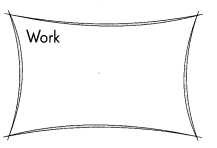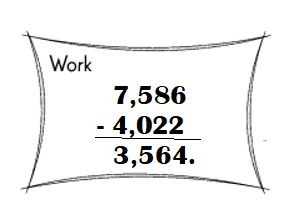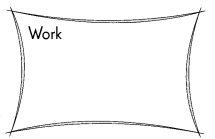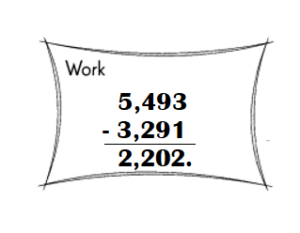# Math in Focus Grade 3 Chapter 4 Practice 1 Answer Key Subtraction Without Regrouping

Go through the Math in Focus Grade 3 Workbook Answer Key Chapter 4 Practice 1 Subtraction Without Regrouping to finish your assignments.

## Math in Focus Grade 3 Chapter 4 Practice 1 Answer Key Subtraction Without Regrouping

Subtract.

Example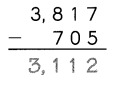Question 1.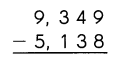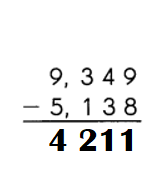Explanation:
9349 – 5138 = 4211.

Question 2.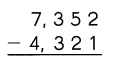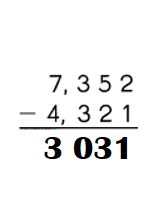Explanation:
7352 – 4321 = 3031.

Example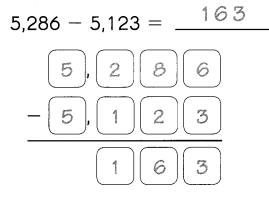Question 3.
3,646 – 2,523 = ____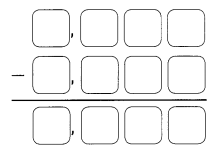3,646 – 2,523 = 1,123.

Explanation: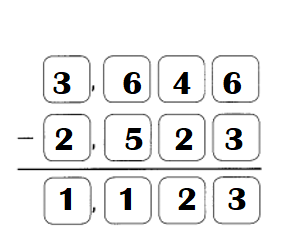Question 4.
9,646 – 523 = ____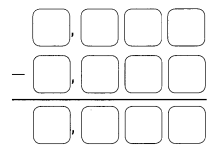9,646 – 523 = 9,123.

Explanation: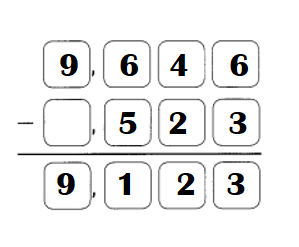Question 5.
5,564 – 23 = ____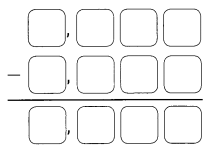5,564 – 23 = 5,541.

Explanation: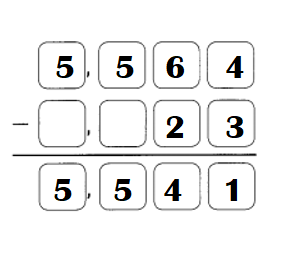Find the difference.

Example
The difference between 7,249 and 249 is 7,000

Question 6.
The difference between 5,286 and 5,000 is ____.
The difference between 5,286 and 5,000 is 286.

Explanation: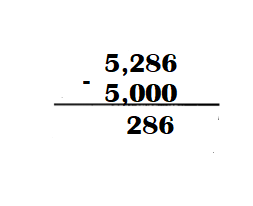Question 7.
The difference between 4,301 and 2,101 is ____.
The difference between 4,301 and 2,101 is 2200.

Explanation: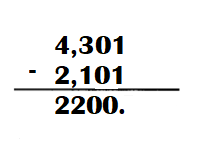Subtract.

Example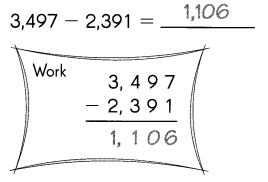Question 8.
8,421 – 310 = ____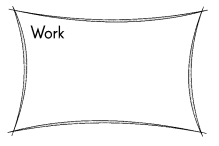8,421 – 310 = 8,111.

Explanation: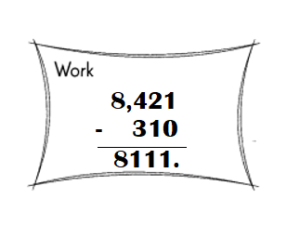Question 9.
9,786 – 72 = ____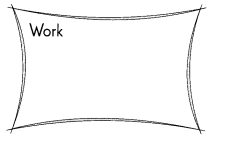9,786 – 72 = 9,714.

Explanation: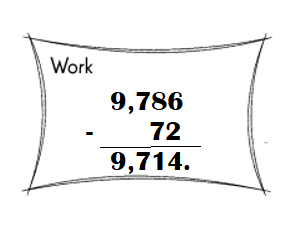Question 10.
6,974 – 1,813 = ____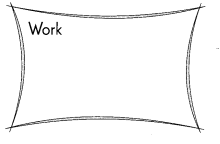6,974 – 1,813 = 5,161.

Explanation: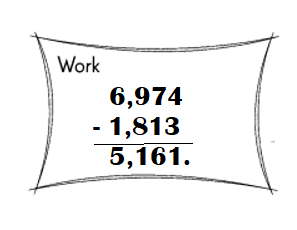Question 11.
7,586 – 4,022 = ___